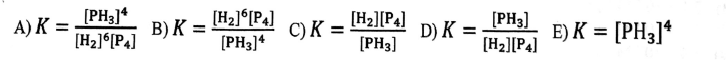Chemistry Practice Problems Equilibrium Expressions Practice Problems Solution: Choose the answer which gives the correct equilibr...

🤓 Based on our data, we think this question is relevant for Professor Del Negro's class at NCSU.

# Solution: Choose the answer which gives the correct equilibrium constant expression for the reaction shown below. 6 H2(g) + P4(g) ⇌ 4 PH3(g)

###### Problem

Choose the answer which gives the correct equilibrium constant expression for the reaction shown below.

6 H2(g) + P4(g) ⇌ 4 PH3(g)Equilibrium Expressions

Equilibrium Expressions

#### Q. Select the correct equilibrium expression for the following reaction.NaH(s) + H2O(l) → NaOH(aq) + H2(g)

Solved • Wed Apr 26 2017 12:05:31 GMT-0400 (EDT)

Equilibrium Expressions

#### Q. Kc = 1.03 x 10-6 for the reaction shown below. if the equilibrium concentrations of H 2 = 0.00463 M and S2 = 0.00684, what is the equilibrium concentr...

Solved • Tue Apr 25 2017 13:56:42 GMT-0400 (EDT)

Equilibrium Expressions

#### Q. Choose the answer which gives the equilibrium expression for the aqueous reaction shown below.Si(OCH3)4(aq) + 2 H2O(l) ⇌ SiO2(s) + 4 HOCH3(aq)

Solved • Tue Apr 18 2017 15:46:37 GMT-0400 (EDT)

Equilibrium Expressions

#### Q. Kc = 2.6 x 108 at 825 K for the reaction2H2(g) + S2(g) ⇌ 2H2S(g)The equilibrium concentration of H2 is 0.0020 M and S2 is 0.0010 M. What is the equili...

Solved • Tue Apr 18 2017 14:48:26 GMT-0400 (EDT)